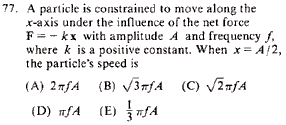GR 8677927796770177 | # Login | Register

GR8677 #77
Problem
 GREPhysics.NET Official Solution Alternate SolutionsWave Phenomena}Wave Equation

The problem gives the equation of motion \par , where .

The general equation for a wave propagating in time and oscillating in the x direction is
. ( is the amplitude, is the angular frequency and is some phase constant.)

This is also the general solution to the differential equation posed above.

Plug in the condition (given by the problem) that \par to get . Recalling the unit circle, the angle \par .

Plug in the argument into the velocity \par
. Recall that , and thus , as in choice (B).Alternate Solutions
ethanque
2015-08-12 08:54:41
Hooke's Law and Work Energy Theorem:

I couldn't remember my wave equations right away so I used the work energy theorem imagining this is a SHO/spring:

The particle has zero kinetic energy when , and it is subject to the force as it travels from to . i.e. the spring does work on the particle.

So, integrate

Recall that . Then

Recall that . Then

, which is option (B).wittensdog
2009-10-07 18:32:43
Yet another solution...

You can easily find that the argument of the sine function must be 1/2. If you know the value of a sine function at one point, you immediately know the value of cosine, since,

cos^2 + sin^2 = 1 ==>

cos = sqrt ( 1 - sin^2 ) ==>

cos = sqrt(3) / 2

which then you just plug right into the velocity formula. It's similar to the original solution, but you don't need to remember what angles correspond to what values of sine and cosine.lattes
2008-08-07 13:08:18
Another to solve is: consider Let and . Squaring both equation and summing them we get: . Now let and solve for . Thus . (you should remember that !). A little bit longer solution, but it works.rreyes
2005-10-31 08:06:24
an alternative solution is conservation of energy

1/2kA^2=1/2kx^2+1/2mv^2

plugging in x=A/2 gives

v= \sqrt{3k/4m}A = \sqrt{3}\pi fA
 yosun2005-11-01 02:14:55 fyi, add in dollar-sign wrappers around the equations in your comments... and the system processes your latex-compatible syntax into nifty-looking equations. quote rreyes: an alternative solution is conservation of energy plugging in gives
 nitin2006-11-16 13:32:49 Thumbs up mate!ethanque
2015-08-12 08:54:41
Hooke's Law and Work Energy Theorem:

I couldn't remember my wave equations right away so I used the work energy theorem imagining this is a SHO/spring:

The particle has zero kinetic energy when , and it is subject to the force as it travels from to . i.e. the spring does work on the particle.

So, integrate

Recall that . Then

Recall that . Then

, which is option (B).

 ethanque2015-08-12 08:58:27 WOW! My solution looked fine in the preview. Sorry, for that jumbled mess of latex jargon... help?
 yosun20152015-08-23 06:22:33 fixed! :)tpatt
2011-09-05 14:08:10
Can someone explain why (omega*t + phi) = pi/6? I'm not following that step.
 livieratos2011-11-08 06:03:21 cause then sin(omega*t + phi) = sin(pi/6) = 1/2 as was given...anum
2010-11-11 11:37:29
v=r*w =2*pi*f*A putting in A/2 in place of A gives v=pi*f* A . but its not correct; can anyone tell why?
 IRFAN 2011-08-18 03:19:30 this velocity you have determin works only at particular point,in next you find the velocity of a different motion wich have halv the amplitrudeshafatmubin
2009-11-02 10:17:59
Simple Harmonic Motion flash card:

x = A cos (wt)
v = w Sqrt(A^2 - x^2)
a = -w^2 x

V = (1/2) k x^2 = (1/2) m w^2 x^2
T = (1/2) m v^2 = (1/2) m w^2 (A^2 - x^2)wittensdog
2009-10-07 18:32:43
Yet another solution...

You can easily find that the argument of the sine function must be 1/2. If you know the value of a sine function at one point, you immediately know the value of cosine, since,

cos^2 + sin^2 = 1 ==>

cos = sqrt ( 1 - sin^2 ) ==>

cos = sqrt(3) / 2

which then you just plug right into the velocity formula. It's similar to the original solution, but you don't need to remember what angles correspond to what values of sine and cosine.
 danielsw986672019-09-30 11:54:45 A very good alternative idea! Nano-Particle CBDlattes
2008-08-07 13:08:18
Another to solve is: consider Let and . Squaring both equation and summing them we get: . Now let and solve for . Thus . (you should remember that !). A little bit longer solution, but it works.
 lattes2008-08-07 13:09:33 "Another way to solve the problem is" ....
 danielsw986672019-09-13 13:04:14 I must try solving it your way. electrical company in jacksonvillerreyes
2005-10-31 08:06:24
an alternative solution is conservation of energy

1/2kA^2=1/2kx^2+1/2mv^2

plugging in x=A/2 gives

v= \sqrt{3k/4m}A = \sqrt{3}\pi fA
 yosun2005-11-01 02:14:55 fyi, add in dollar-sign wrappers around the equations in your comments... and the system processes your latex-compatible syntax into nifty-looking equations. quote rreyes: an alternative solution is conservation of energy plugging in gives
 nitin2006-11-16 13:32:49 Thumbs up mate!
 zeper2013-03-24 11:59:00 that is the best one....;)LaTeX syntax supported through dollar sign wrappers $, ex.,$\alpha^2_0$produces . type this... to get...$\int_0^\infty\partial\Rightarrow\ddot{x},\dot{x}\sqrt{z}\langle my \rangle\left( abacadabra \right)_{me}\vec{E}\frac{a}{b}\$